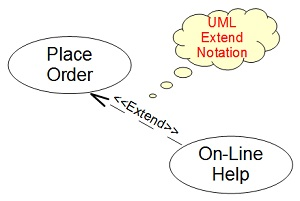Use Case Diagram - Extend Notation

This section describes the Extend Notation used in a UML Use Case Diagram. An Extend Notation presents an extension relation between two use cases where one acts as the base use case and the other acts as an add-on use case.

An Extend Notation is a graphical notation used in a UML Use Case Diagram to represent an extension relation between two use cases where one acts as the base use case and the other acts as an add-on use case. The base use case is usually called the Extended Use Case. The add-on use case is usually called the Extending Use Case.

An Extend Notation is usually drawn as a dash line with a line arrow connecting both use cases. The arrow of an Extend Notation should point to the extended use case. An Extend Notation should also be labeled with the "<<extend>>" key word.

For example, the "On-Line Help" use case is an extension to the "Place Order" use case. This extension relation can be drawn as an Extend Notation in a UML use case diagram as shown below:Last update: 2014.